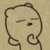数组合并，怎么样才比较快

yangrfa 发布于 01/17 14:29

【推荐阅读】滴滴出行的数据架构和信息流处理思路解析->>>1. 如果a1(x=1, y=3), a2(x=3, y=4), a3(x=4, y=7)，这三个元素可以合并成一个新的二元组c(x=1, y=7)，即前一个元素的y和后一个的x相同，可以合并；

2. 如果a1(x=1, y=2), a2(x=3, y=4), a3(x=4, y=7)，合并后的结果是c1(x=1, y=2), c2(x=3, y=7)，即前一个元素的y和后一个的x不相同，不能合并；

3. 如果a1(x=1, y=2), a2(x=3, y=4), a3(x=5, y=7)，则三个都不能合并，结果是c1(x=1, y=2), c2(x=3, y=4), c3(x=5, y=7)

0x y 分成两个数组，相同的数两数组都去除，最终结果就是一个x 一个y，一个x一个y 。。。。